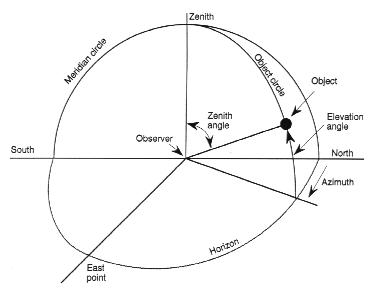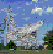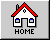Horizon Coordinate System

The horizon is defined as the dividing line between the Earth and the sky, as seen by an observer on the ground. In the horizon coordinate system the astronomical horizon is the hypothetical interface between Earth and sky, as would be seen by the observer if the surrounding terrain were perfectly flat (as out on a calm ocean).

Referring to the drawing below, zenith is the point straight overhead, perpendicular to the horizon plane, and nadir is the point directly under the observer. A vertical circle through an object in the sky and the zenith is the object circle. The coordinates of the object are given by the azimuth, which is the horizontal angle from north clockwise to the object circle, and the altitude or elevation angle, which is measured upward from the horizon to the object. The great circle through the north and south points on the horizon and the zenith is called the meridian.

Horizon Coordinate SystemA horizon mask is a diagram that maps in silhouette the horizon in 360° of azimuth as actually seen by the observer, including hills, valleys, mountains, buildings, trees, and anything else that would hide from view any part of the sky that would be visible if the terrain were perfectly flat.

In the horizon system, the coordinates of an object in the sky change throughout the day with Earth’s rotation. While the azimuth and elevation angles are convenient for positioning a radio telescope antenna that rotates around horizontal and vertical axes (AZ-EL mounted), they are not so convenient for specifying the position of a celestial object. Better for this purpose are systems using fixed coordinates, such as the equatorial coordinate system.

GBT Operations index